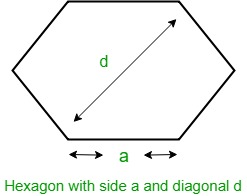# Area of hexagon with given diagonal length

You are given the length of the diagonal of a hexagon, d. Your task is to find the area of that hexagon.

Examples:

Input :   5
Output :   Area of Hexagon: 16.238

Input : 10
Output : Area of Hexagon: 64.9519


Hexagon
Hexagon is a regular polygon having six equal sides and all equal angles. The interior angles of Hexagon are of 120 degrees each and the sum of all angles of a Hexagon is 720 degrees.Let d be the diagonal of Hexagon, then the formula to find the area of Hexagon given by
Area =How does above formula work?
We know that area of hexagon with side length a = (3 √3(a)2 ) / 2. Since all sides are of same size and angle is 120 degree, d = 2a or a = d/2. After putting this value, we get area as (3 √3(d)2 ) / 8.
Below is the implementation .

## C++

 // C++ program to find the area of Hexagon with given diagonal  #include  using namespace std;     // Function to calculate area  float hexagonArea(float d)  {      // Formula to find area      return (3 * sqrt(3) * pow(d, 2)) / 8;  }     // Main  int main()  {      float d = 10;      cout << "Area of hexagon: " << hexagonArea(d);      return 0;  }

## Java

 // Java program to find the area of   // Hexagon with given diagonal   import java.lang.Math;      public class GfG {         // Function to calculate area       public static float hexagonArea(float d)       {           // Formula to find area           return (float)((3 * Math.sqrt(3) * d * d) / 8);       }          public static void main(String []args) {          float d = 10;          System.out.println("Area of hexagon: " + hexagonArea(d));      }  }     // This code is contributed by Rituraj Jain

## Python3

 # Python3 program to find the area   # of Hexagon with given diagonal   from math import sqrt     # Function to calculate area   def hexagonArea(d) :         # Formula to find area       return (3 * sqrt(3) * pow(d, 2)) / 8    # Driver ode  if __name__ == "__main__" :          d = 10     print("Area of hexagon:",              round(hexagonArea(d), 3))     # This code is contributed by Ryuga

## C#

 // C# program to find the area of   // Hexagon with given diagonal      using System;     public class GFG{             // Function to calculate area       public static float hexagonArea(float d)       {           // Formula to find area           return (float)((3 * Math.Sqrt(3) * d * d) / 8);       }         //Code driven      static public void Main (){              float d = 10;          Console.WriteLine("Area of hexagon: " + hexagonArea(d));      }  //This code is contributed by Tushil.      }

## PHP

 

Output:

Area of Hexagon:  64.952

Time Complexity: O(1)

Attention reader! Don’t stop learning now. Get hold of all the important DSA concepts with the DSA Self Paced Course at a student-friendly price and become industry ready.

My Personal Notes arrow_drop_upCheck out this Author's contributed articles.

If you like GeeksforGeeks and would like to contribute, you can also write an article using contribute.geeksforgeeks.org or mail your article to contribute@geeksforgeeks.org. See your article appearing on the GeeksforGeeks main page and help other Geeks.

Please Improve this article if you find anything incorrect by clicking on the "Improve Article" button below.

Improved By : AnkitRai01, rituraj_jain, jit_t